If there is one prayer that you should pray/sing every day and every hour, it is the LORD's prayer (Our FATHER in Heaven prayer)
It is the most powerful prayer. A pure heart, a clean mind, and a clear conscience is necessary for it.
- Samuel Dominic Chukwuemeka

The Joy of a Teacher is the Success of his Students. - Samuel Chukwuemeka

# Chemical Reactions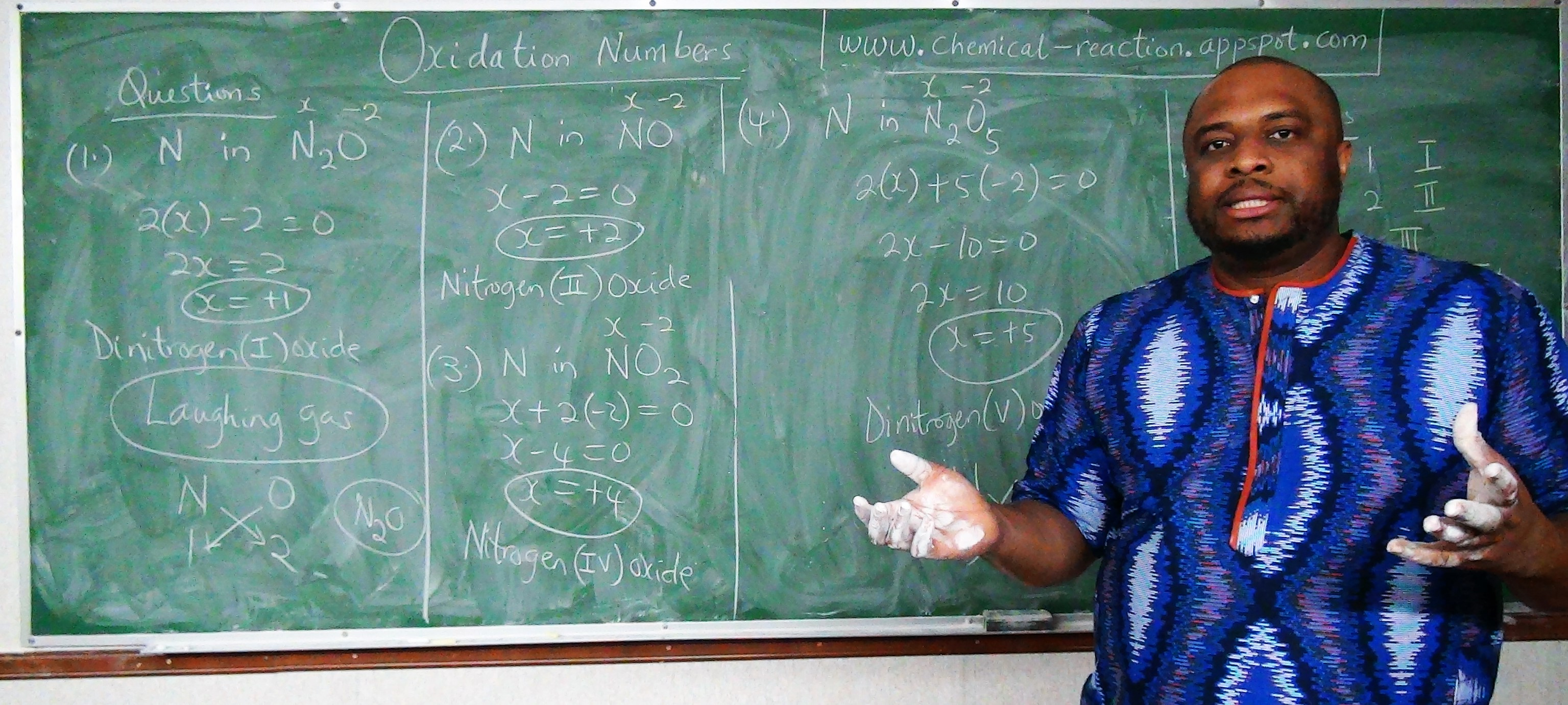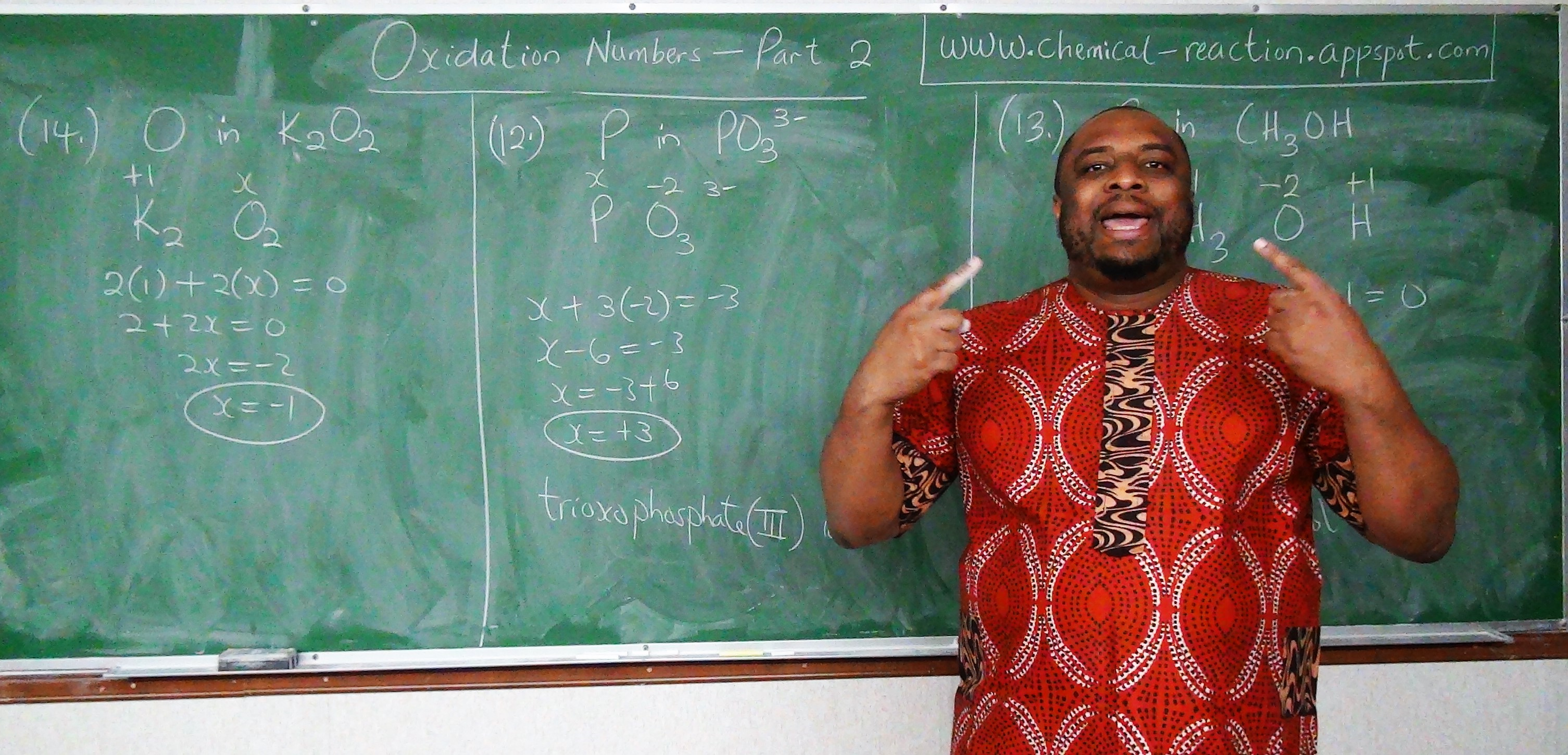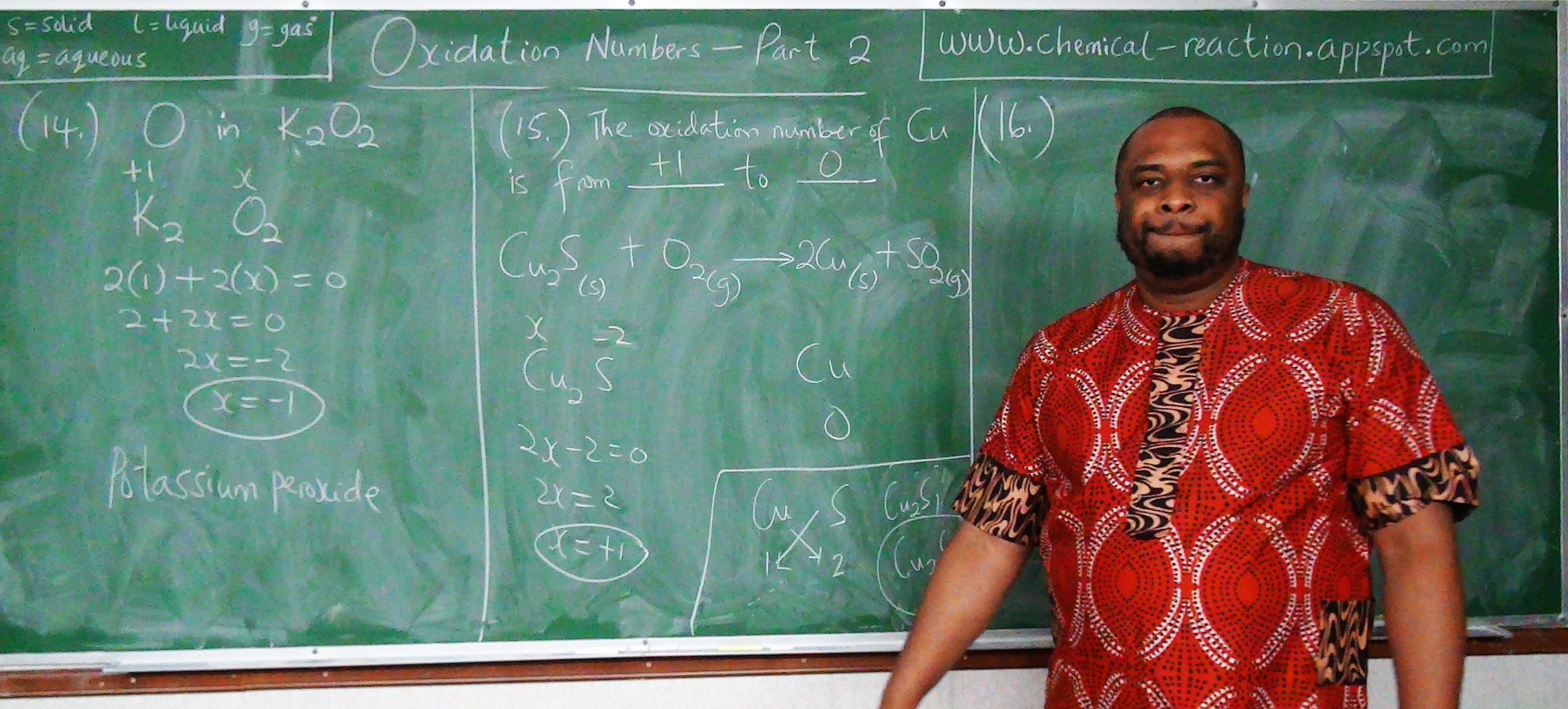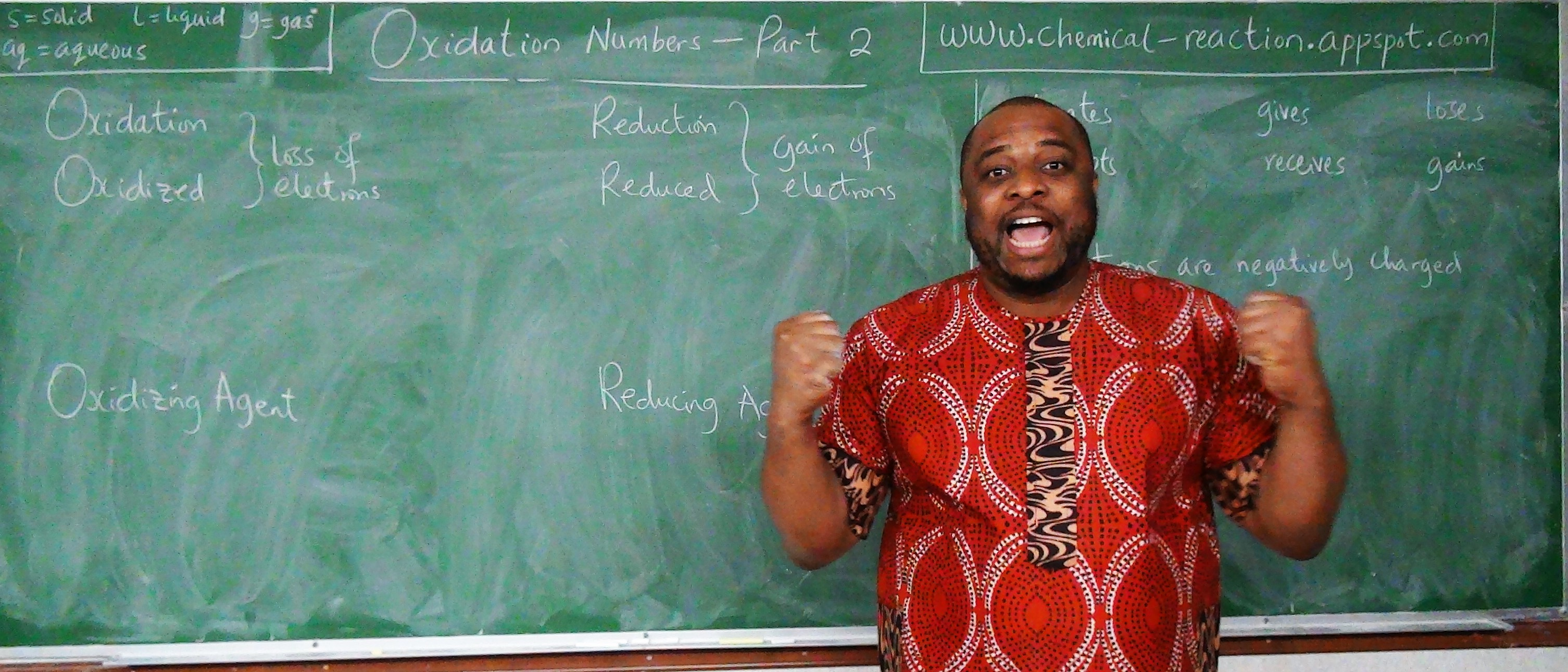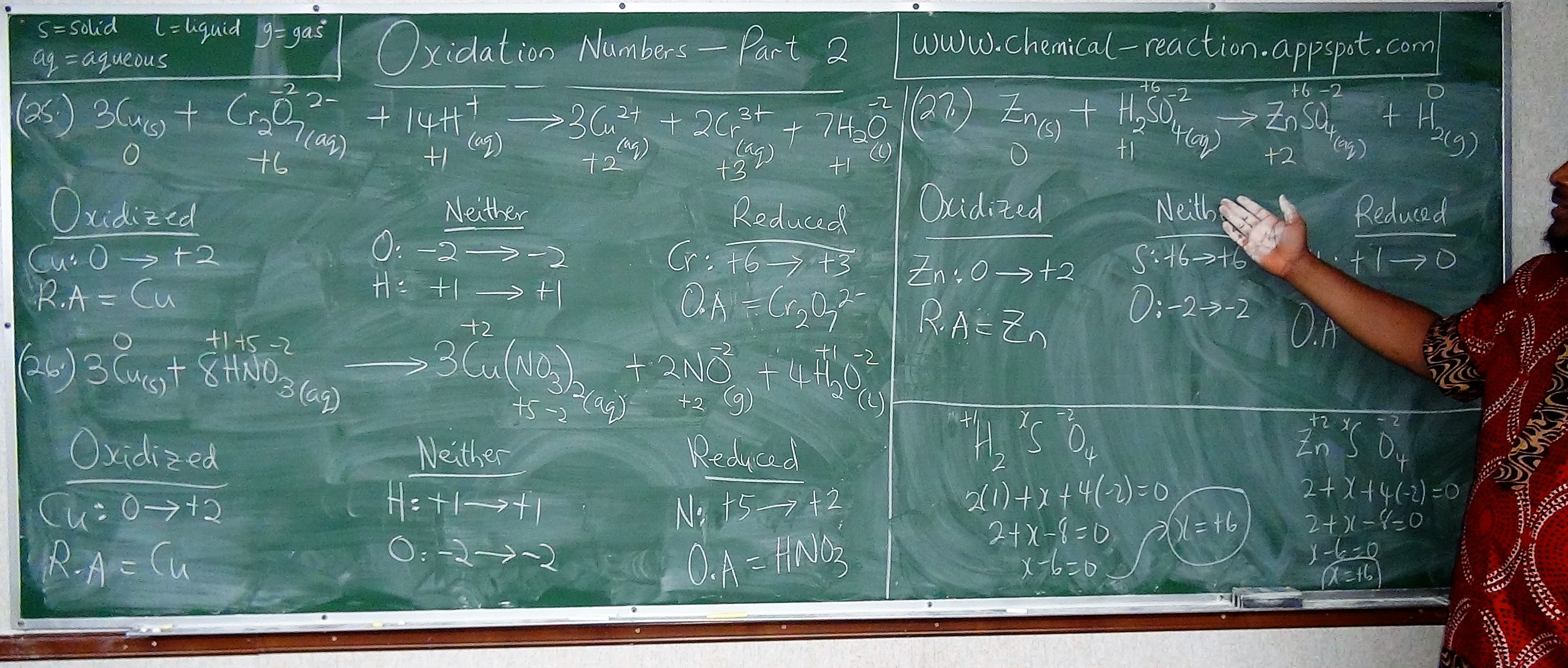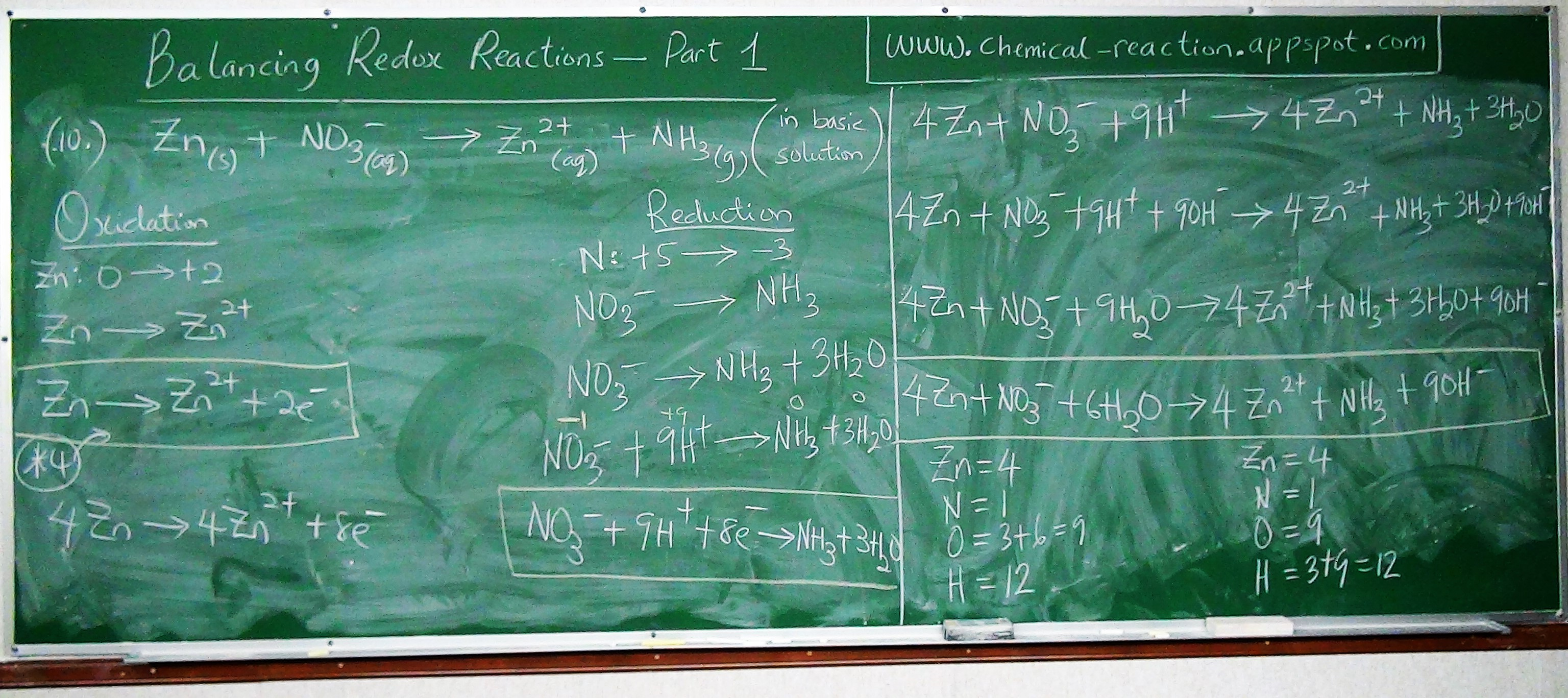I greet you this day,
Second: view the videos.
Third: solve the questions/solved examples.
Fourth: check your solutions with my thoroughly-explained solutions.
Fifth: check your answers with the calculators as applicable. Many thanks to the Wolfram Alpha Widget developers.
Comments, ideas, areas of improvement, questions, and constructive criticisms are welcome. You may contact me.
If you are my student, please do not contact me here. Contact me via the school's system. Thank you for visiting.

Samuel Dominic Chukwuemeka (SamDom For Peace) B.Eng., A.A.T, M.Ed., M.S

## Objectives

Students will:

(1.) Define a chemical reaction.
(2.) Write the states of the reactants of a chemical reaction.
(3.) Write the states of the products of a chemical reaction.
(4.) Predict the products of a chemical reaction, given the reactants of the reaction.
(5.) Balance chemical reactions using the Inspection Method.
(6.) Balance chemical reactions using the Algebraic Method.
(7.) List the types of chemical reactions.
(8.) Discuss Combination (or Synthesis) reactions.
(9.) Discuss Decomposition reactions.
(10.) Discuss Thermal Decomposition reactions.
(11.) Discuss Displacement (or Single Displacement or Single Replacement) reactions.
(12.) Discuss Double Displacement (or Metathesis) reactions.
(13.) Discuss Double Decomposition (or Precipitation) reactions.
(14.) Discuss Catalytic reactions.
(15.) Discuss Reversible reactions.
(16.) Discuss Thermal Dissociation reactions.
(17.) Discuss Oxidation-Reduction (or Redox) reactions.
(18.) Discuss Combustion reactions.

## Vocabulary Words

Bring it to English: Prefixes and Meanings:
mono = 1, di = 2, tri = 3, tetra = 4, penta = 5, hexa = 6, hepta = 7, octa = 8, nona = 9, deca = 10; etc.
This implies that $tri-oxo$ means $3$ oxygen atoms; $di-chromate$ means 2 chromium atoms; etc.
solid, liquid, gas, aqueous

## Algebraic Method for Balancing Chemical Reactions

To balance chemical reactions using the Algebraic Method:

(1.) Make sure that the reactant(s) and product(s) are correct.

(2.) Make sure the chemical formulas for the reactant(s) and product(s) are correct.

(3.) Put variables in front of all the reactant(s).

(4.) Put variables in front of every product besides one product.
If there is one product, do not put a variable.
If there are two products, put a variable in front of only one product. You can put a variable in either one of them. It does not matter.
If there are three products, put variables in front of any of the two products.
If there are four products, put variables in front of any of the three products.

Let us do an example.
Write a balanced reaction between tetraoxosulphate($VI$)acid with aluminium hydroxide to give water and aluminium tetraoxosulphate($VI$)

$Valency\:\:of\:\:Al = 3 \\[3ex] Valency\:\:of\:\:SO_4 = 2 \\[3ex] \therefore Al_{2}(SO_4)_3 \\[3ex] Valency\:\:of\:\:Al = 3 \\[3ex] Valency\:\:of\:\:OH = 1 \\[3ex] \therefore Al(OH)_3 \\[3ex] Valency\:\:of\:\:H = 1 \\[3ex] Valency\:\:of\:\:SO_4 = 2 \\[3ex] \therefore H_{2}SO_4 \\[3ex] Valency\:\:of\:\:H = 1 \\[3ex] Valency\:\:of\:\:O = 2 \\[3ex] \therefore H_{2}O \\[3ex]$ The unbalanced reaction is:
$H_{2}SO_4 + Al(OH)_3 \rightarrow Al_{2}(SO_4)_3 + H_{2}O$

Teacher: So, you have an acid reacting with a base to give a salt and water
What do we call this type of reaction?

$Acid + Base \rightarrow Salt + Water$ is a Neutralization Reaction

Let us balance the reaction using the Algebraic method
We have two reactants and two products
We shall place two different variables in front of the two reactants and one variable in front of one product.

$mH_{2}SO_4 + nAl(OH)_3 \rightarrow pAl_{2}(SO_4)_3 + H_{2}O \\[3ex] H = 2m + 3n ~~~~~\:\:\:\:\:\:\: H = 2 \\[3ex] S = m ~~~~~~~~~~~~~~~~~\:\:\:\:\:\:\: S = 3p \\[3ex] O = 4m + 3n ~~~~~\:\:\:\:\:\:\: O = 12p + 1 \\[3ex] Al = n ~~~~~~~~~~~~~~~\:\:\:\:\:\:\: Al = 2p \\[3ex] 2m + 3n = 2 ...eqn.(1) \\[3ex] m = 3p ...eqn.(2) \\[3ex] 4m + 3n = 12p + 1 ...eqn.(3) \\[3ex] n = 2p ...eqn.(4) \\[3ex] Substitute\:\:eqn.(2)\:\:and\:\:eqn.(4)\:\:in\:\:eqn.(1) \\[3ex] Substitute\:\:3p\:\:for\:\:m\:\:and\:\:2p\:\:for\:\:n\:\:in\:\:eqn.(1) \\[3ex] From\:\:eqn.(1)\:\: 2(3p) + 3(2p) = 2 \\[3ex] 6p + 6p = 2 \\[3ex] 12p = 2 \\[3ex] p = \dfrac{2}{12} \\[5ex] p = \dfrac{1}{6} \\[5ex] Substitute\:\:the\:\:value\:\:of\:\:p\:\:in\:\:eqn.(2) \\[3ex] From\:\:eqn.(2)\:\: m = 3\left(\dfrac{1}{6}\right) \\[5ex] m = \dfrac{1}{2} \\[5ex] Substitute\:\:the\:\:value\:\:of\:\:p\:\:in\:\:eqn.(4) \\[3ex] From\:\:eqn.(4)\:\: n = 2\left(\dfrac{1}{6}\right) \\[5ex] m = \dfrac{1}{3} \\[5ex] Substitute\:\:the\:\:values\:\:back\:\:in\:\:the\:\:equation \\[3ex] \dfrac{1}{2}H_{2}SO_4 + \dfrac{1}{3}Al(OH)_3 \rightarrow \dfrac{1}{6}Al_{2}(SO_4)_3 + H_{2}O \\[5ex] LCD = 6 \\[3ex] Multiply\:\:each\:\:reactant\:\:and\:\:each\:\:product\:\:by\:\:the\:\:LCD \\[3ex] 6 * \dfrac{1}{2}H_{2}SO_4 + 6 * \dfrac{1}{3}Al(OH)_3 \rightarrow 6 * \dfrac{1}{6}Al_{2}(SO_4)_3 + 6 * 1H_{2}O \\[5ex] \therefore 3H_{2}SO_4 + 2Al(OH)_3 \rightarrow Al_{2}(SO_4)_3 + 6H_{2}O \\[3ex]$

Check by Inspection Method
 $\underline{Reactants} \\[3ex] H = 3 * 2 + 2 * 3 = 6 + 6 = 12 \\[3ex] S = 3 \\[3ex] O = 3 * 4 + 2 * 3 = 12 + 6 = 18 \\[3ex] Al = 2$ $\underline{Products} \\[3ex] H = 6 * 2 = 12 \\[3ex] S = 3 \\[3ex] O = 4 * 3 + 6 = 12 + 6 = 18 \\[3ex] Al = 2$

For more examples, please review: Solved Examples on the Balancing of Chemical Reactions using the Algebraic Method

## Calculator for Chemical Reactions

This calculator will:
(1.) Give the product(s) of an unbalanced chemical reaction when given the reactant(s)
(2.) Balance a chemical reaction when given the reactant(s) and the product(s)

To use the calculator:
(1.) If you want to determine the products of a chemical reaction:
1st: Type only the reactants and the arrow in the big textbox
The arrow is the hyphen and the greater than symbol on your keyboard: ->
2nd: Copy and paste it in the small textbox (actual calculator)
You may also type directly in the small textbox if you wish. The big textbox is just for you to see everything you typed, before you copy and paste it into the small textbox.
The small textbox is the actual calculator.

3rd: Click the Submit button
4th: Review the Sample reactions in the output.
You may need to click the Show formulas link on the RHS (Right Hand Side) to see the chemical reaction(s).
Those are the likely products of the chemical reaction.
They are not balanced. So, you will need to copy any one you want to balance and read on to Number (2.)

(2.) If you want to balance any chemical reaction:
1st: Type the reactants, then the arrow, then the products in the big textbox
The arrow is the hyphen and the greater than symbol on your keyboard: ->
2nd: Copy and paste it in the small textbox (actual calculator)
You may also type directly in the small textbox if you wish. The big textbox is just for you to see everything you typed, before you copy and paste it into the small textbox.
The small textbox is the actual calculator.

3rd: Click the Submit button
4th: Review the balanced chemical reaction in the output.

• See Examples (based on these solved examples: Balance Chemical Reactions)
• To determine the products of a reaction, Type: $SO_2 + O_2 \rightarrow$ as SO2 + O2 ->
• To balance the reaction, Type: $SO_2 + O_2 \rightarrow SO_3$ as SO2 + O2 -> SO3
• To determine, Type: $C_4H_{10} + O_2 \rightarrow$ as C4H10 + O2 ->
• To balance, Type: $C_4H_{10} + O_2 \rightarrow CO_2 + H_2O$ as C4H10 + O2 -> CO2 + H2O
• To determine, Type: $Cu + HNO_3 \rightarrow$ as Cu + HNO3 ->
• To balance, Type: $Cu + HNO_3 \rightarrow Cu(NO_3)_2 + NO + H_2O$ as Cu + HNO3 -> Cu(NO3)2 + NO + H2O
• To determine, Type: $Fe(OH)_3 + H_2SO_4 \rightarrow$ as Fe(OH)3 + H2SO4 ->
• To balance, Type: $Fe(OH)_3 + H_2SO_4 \rightarrow Fe_2(SO_4)_3 + H_2O$ as Fe(OH)3 + H2SO4 -> Fe2(SO4)3 + H2O

Type:

## Oxidation Number

Oxidation number is also known as Oxidation state.
The oxidation number of an element is the number of electrons the element gains or loses in a chemical combination with another element or compound.
Generally; metals have positive oxidation numbers (because they donate electrons), and non-metals have negative oxidation numbers (because they accept electrons).
Be it as it may, some elements have several oxidation numbers. It just depends on the chemical formula.
Recall: When elements react, there is a transfer of electrons (electrovalent bonding), or sharing of electrons (covalent bonding).
When an element donates (loses) an electron or receives (gains) an electron, it becomes an ion.
Metals are electron donors, while non-metals are electron acceptors.
The oxidation number can be positive, zero, or negative.
A substance can be an atom, element, compound, molecule, or an ion.

### Why Study Oxidation Numbers?

Oxidation numbers helps us to:
(1.) Name compounds according to the IUPAC (International Union of Pure and Applied Chemistry) nomenclature.
(2.) Balance reduction-oxidation (redox) reactions.

### Rules for Assigning Oxidation Numbers

(1.) The oxidation number of any element in its free state (when it is not combined with any substance) is 0.
Recall: Some elements are monoatomic or polyatomic in their free states.
Example:
The oxidation numbers of monoatomic $Na$ (sodium), diatomic $O_2$ (oxygen), triatomic $O_3$ (ozone), tetra-atomic $P_4$ (phosphorus), and octa-atomic $S_8$ (sulphur) among others is 0.

(2.) The oxidation number of any compound is 0.
This applies to binary, ternary, and tetranary compounds among others.
Recall: A binary compound is a compound made up of two elements. A ternary compound is a compound made up of three elements. A tetranary or quaternary compound is a compound made up of four elements.
Example:
The oxidation numbers of the binary compound $CuCl_2$ (copper (II) chloride), ternary compound $KMnO_4$ (potassium tetra-oxo manganate (VII) - also known as potassium permanganate), and the tetranary compound $NaHCO_3$ (sodium hydrogen tri-oxo carbonate (IV) - also known as baking soda) is 0.
Do you wonder how I got the roman numerals: II, VII, IV? Do you know what they mean?
The oxidation number of copper in copper (II) chloride is 2.
The oxidation number of manganese in potassium tetra-oxo manganate (VII) is 7.
The oxidation number of carbon in sodium hydrogen tri-oxo carbonate (IV) is 4.
Is it making a little sense? As you can see, the oxidation number helps us in naming compounds according to the IUPAC naming system.

(3.) The sum of the oxidation numbers of each individual element in a compound is equal to the oxidation number of the compound.
Because the oxidation number of any compound is 0, this implies that the sum of the oxidation numbers of each element in a compound is 0.
Example:
The oxidation number of $CuCl_2 = 0$
This means that the sum of the oxidation numbers of copper and chlorine is zero.
We already know from the IUPAC nomenclature that the oxidation number is +2.
With that knowledge, we can find the oxidation number of chlorine in copper (II) chloride.
Let the oxidation number of $Cl$ in $CuCl_2 = x$
$Cu = 2$
$Cl_2 = 2 * x = 2x$
$CuCl_2 = 0$
$\therefore 2 + 2x = 0$
$2x = 0 - 2$
$2x = -2$
$x = -1$
This means that the oxidation number of chlorine in copper (II) chloride is $-1$.
Do you see the usefulness of this $3^{rd}$ rule?

(4.) The oxidation number of a monoatomic ion is the charge on the ion.
Recall: A monoatomic ion is an ion formed from one atom.
Examples:
The oxidation number of aluminium, $Al$ in the aluminium ion, $Al^{3+}$ is $+3$.
The oxidation number of selenium, $Se$ in the selenium ion (known as selenide), $Se^{2-}$ is $-2$.

(5.) The oxidation number of a polyatomic ion is the charge on the ion.
Recall: A polyatomic ion is an ion formed from more than one atom.
This implies that the sum of the oxidation numbers of each atom in the polyatomic ion is equal to the charge on the ion. Example:
In this polyatomic ion, $Cr_2O_7^{2-}$ (Can you name the ion? ☺☺☺); .
The sum of the oxidation numbers of $Cr$ and $O$ is $-2$
Say the oxidation number of oxygen is $-2$, we can find the oxidation number of chromium
Let the oxidation number of chromium = $x$
$Cr = 2 * x = 2x$
$O = 7 * -2 = -14$
$Cr_2O_7^{2-} = -2$
$\therefore 2x + (-14) = -2$
$2x - 14 = -2$
$2x = -2 + 14$
$2x = 12$
$x = +6$
This means that the oxidation number of chromium in the ion is $+6$
Ask students to try and name the ion according to IUPAC nomenclature
The ion is the hepta-oxo dichromate(VI) ion.

(6.) The oxidation number of an alkali metal in any compound is always $+1$.
Recall: Alkali metals are the elements in Group 1A of the periodic table.
They include: lithium $Li$, sodium $Na$, potassium $K$, rubidium $Rb$, cesium $Cs$, and Francium $Fr$. Example:
The oxidation number of sodium in any compound of sodium is always $+1$.
So, the oxidation number of $Na$ in $NaHCO_3$ is $+1$.

(7.) The oxidation number of an alkaline earth metal in any compound is always $+2$.
Recall: Alkaline earth metals are the elements in Group 2A of the periodic table.
They include: beryllium $Be$, magnesium $Mg$, calcium $Ca$, strontium $Sr$, barium $Ba$, and Radium $Ra$. Example:
The oxidation number of calcium in any compound of calcium is always $+2$.
So, the oxidation number of $Ca$ in $Ca_3(PO_4)_2$ is $+2$.

(8.) The oxidation number of Fluorine in any compound is always $-1$.
Examples:
The oxidation number of $F$ in Bismuth Fluoride, $BiF_3$ is $-1$.
The oxidation number of $F$ in Silicon Fluoride (Silicon tetrafluoride), $SiF_4$ is $-1$.

(9.) The oxidation number of hydrogen in any compound is usually $+1$, except in binary metallic hydrides.
The oxidation number of hydrogen in binary metallic hydrides is $-1$.
Recall: A binary metallic hydride is a compound of a metal and hydrogen.
Examples:
The oxidation number of hydrogen in Ammonia, $NH_3$ is $+1$. Nitrogen is not a metal. So, $NH_3$ is not a metallic hydride.
But, the oxidation number of hydrogen in Lithium Hydride, $LiH$ is $-1$. Lithium is a metal. So, $LiH$ is a metallic hydride.

(10.) The oxidation number of oxygen in any compound is usually $-2$; except in peroxides, or if it is bonded with fluorine.
The oxidation number of oxygen in peroxides is $-1$.
The oxidation number of oxygen in compounds of oxygen and fluorine (oxygen fluorides) varies.
However, because we know the oxidation number of fluorine ($-1$), we can calculate the oxidation number of oxygen.
Recall: A peroxide is a compound that contains two oxygen atoms joined together by a single bond.
Examples:
The oxidation number of oxygen in Hydrogen Peroxide, $H_2O_2$ is $-1$.

For the oxygen fluorides, let us do some examples.
What is the oxidation number of oxygen in Oxygen difluoride, $OF_2$?
Let the oxidation state of oxygen be $x$
We already know from Rule (8.) to be $-1$
$x + 2(-1) = 0$
$x - 2 = 0$
$x = +2$

What is the oxidation number of oxygen in dioxygen difluoride, $O_2F_2$?
Let the oxidation state of oxygen be $x$
We already know from Rule (8.) to be $-1$
$2x + 2(-1) = 0$
$2x - 2 = 0$
$2x = 2$
$x = +1$
As you see, oxygen has various oxidation states when it is bonded with fluorine.

(11.) The oxidation number of chlorine $Cl$, bromine $Br$, and iodine $I$ in any compound is usually $-1$, except in combination with oxygen or fluorine.
When either of these elements combine with oxygen or fluorine, we can find their oxidation numbers because we know the oxidation state of oxygen, $-2$ and fluorine, $-1$.
Examples:
What is the oxidation number of chlorine in Sodium chloride (also known as your common salt), $NaCl$?
Let the oxidation state of chlorine be $x$
We already know from Rule (6.) that the oxidation number of sodium is $+1$
$1 + x = 0$
$x = -1$

What is the oxidation number of chlorine in Dichlorine heptoxide, $Cl_2O_7$?
Let the oxidation state of chlorine be $x$
$2x + 7(-2) = 0$
$2x - 14 = 0$
$2x = 14$
$x = +7$
Ask students to rename the compound according to the IUPAC nomenclature.
The old name is Dichlorine heptoxide
The IUPAC name is Chlorine (VII) oxide

(12.) For all other elements not mentioned in Rules (1.) through (9.):
If you were not asked to calculate their oxidation numbers, then assume their oxidation numbers to be their valencies (as determined by their electronic configurations in shells, not orbitals).
Recall: The valency of an element is the combining power of the element.

### Can we have fractional oxidation numbers?

Ask students to name this compound, $Fe_3O_4$ the common way - with prefixes.
Then, ask them to calculate the oxidation number of $Fe$ in $Fe_3O_4$.

The common name for $Fe_3O_4$ is Triiron tetraoxide
We know from Rule (10.) that the oxidation number of oxygen is $-2$
Let the oxidation number of iron be $x$
$3(x) + 4(-2) = 0$
$3x - 8 = 0$
$3x = 8$
$x = {8\over3}$
Hmmm...what happended? This is a fraction! Let me go back to the definition of an oxidation number.
The number of electrons cannot be a fraction. It has to be an integer!
Your students are correct if they make this argument.

Here is the explanation:
When we say the oxidation number of $Ca$ in $Ca_3(PO_4)_2$ is $+2$;
We mean that the oxidation number of a calcium atom is $+2$
We do realize that we have $3$ calcium atoms in the compound, $Ca_3(PO_4)_2$
But, we found the oxidation number of $1$ calcium atom.
If we have to find the oxidation number of 3 calcium atoms, then it would be $3(+2) = +6$
So, we have been finding the oxidation numbers of single ($1$) atoms of elements all along.
Usually, atoms of the same element in a compound have the same oxidation number.
As you can see, the $3$ calcium atoms each have the same oxidation number of $+2$
However, it is possible for atoms of the same element in a compound to have different oxidation numbers.
So, in the case of $Fe_3O_4$;
We have $3$ atoms of Iron
Each of the two of the iron atoms has an oxidation number of $+3$ while the other iron atom has an oxidation number of $+2$
This is known as Fragmenting.
The oxidation number of the 3 iron atoms is $+8$
From our calculation, we got the oxidation number of an iron atom to be ${8\over3}$
As you can notice, this is an average.
So, you can say that the oxidation numbers we have been calculating are averages.
In essence; oxidation numbers cannot be fractions, because the number of electrons donated or accepted cannot be fractions.
So, if you get fractions and you are sure you followed the rules; then you may need to apply fragmenting to know the oxidation states of each atom.
Some students may ask you how you fragment - how do you know that each of the two iron atoms had oxidation number of $+3$ while the other iron atom had an oxidation number of $+2$
Please be prepared to answer. Just tell them it is beyond the scope of this topic.
If they ask whether it is acceptable to write the oxidation number of $Fe$ in $Fe_3O_4$ as ${8\over3}$, let them know they can do so.
What if they ask you to name the compound according to IUPAC nomenclature?
$Fe_3O_4$ is Iron (II, III) oxide.
It is an ionic compound whose atoms have different oxidation numbers.
One atom of iron (II), $Fe^{2+}$ has an oxidation number of $+2$
Two atoms of iron (III), $Fe^{3+}$ each has an oxidation number of $+3$
Hence, the Iron (II, III) oxide